o
osc_ogi0qclx

## 2、占据栅格地图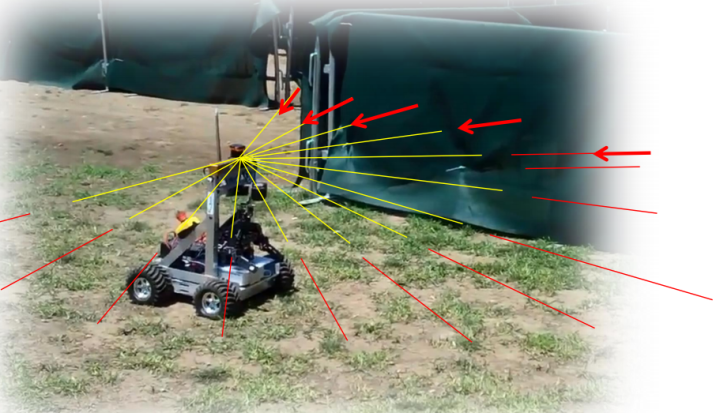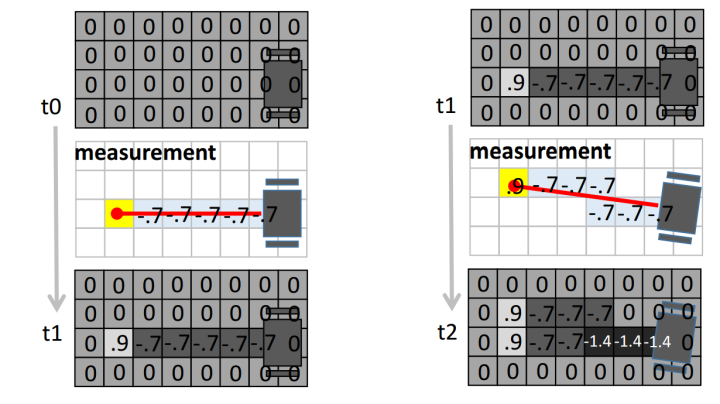## 3. 利用激光传感器构建占据栅格地图

``````function myMap = occGridMapping(ranges, scanAngles, pose, param)
``````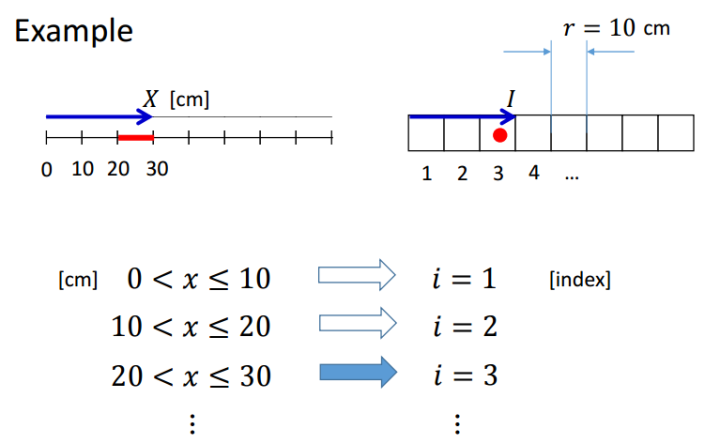ceil向上取整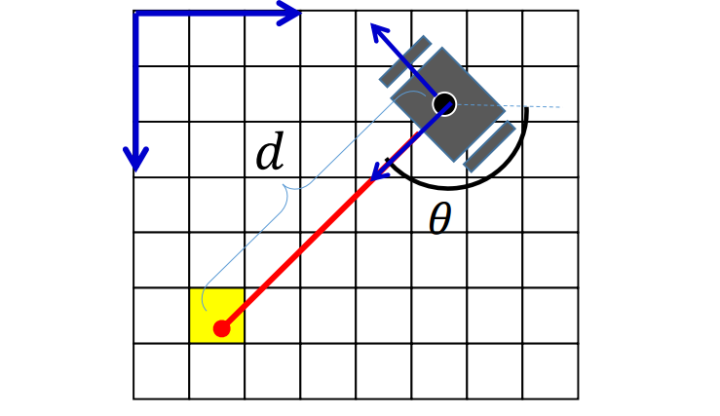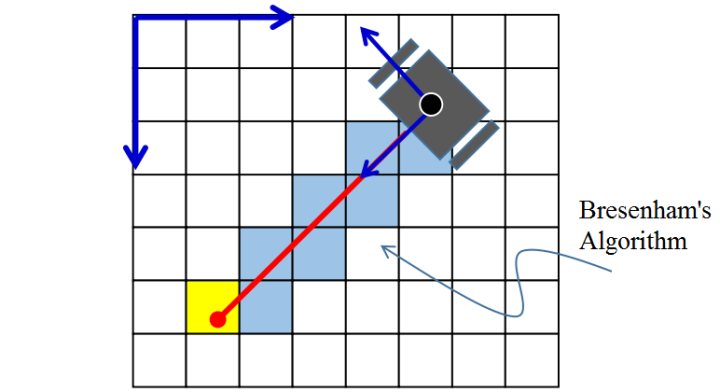``function myMap = occGridMapping(ranges, scanAngles, pose, param)  resol = param.resol; % the number of grids for 1 meter. myMap = zeros(param.size); % the initial map size in pixels origin = param.origin; % the origin of the map in pixels % Log-odd parameters lo_occ = param.lo_occ; lo_free = param.lo_free; lo_max = param.lo_max; lo_min = param.lo_min; lidarn = size(scanAngles,1); % number of rays per timestamp N = size(ranges,2); % number of timestamp for i = 1:N % for each timestamp theta = pose(3,i); % orientation of robot % coordinate of robot in real world x = pose(1,i); y = pose(2,i); % local coordinates of occupied points in real world local_occs = [ranges(:,i).*cos(scanAngles+theta), -ranges(:,i).*sin(scanAngles+theta)]; % coordinate of robot in metric map grid_rob = ceil(resol * [x; y]); % calc coordinates of occupied and free points in metric map for j=1:lidarn real_occ = local_occs(j,:) + [x, y]; % global coordinate of occ in real world grid_occ = ceil(resol * real_occ); % coordinate of occ in metric map % coordinates of free in metric map (by breshnham's algorithm) [freex, freey] = bresenham(grid_rob(1),grid_rob(2),grid_occ(1),grid_occ(2)); % convert coordinate to offset to array free = sub2ind(size(myMap),freey+origin(2),freex+origin(1)); occ = sub2ind(size(myMap), grid_occ(2)+origin(2), grid_occ(1)+origin(1)); % update metric map myMap(free) = myMap(free) - lo_free; myMap(occ) = myMap(occ) + lo_occ; end end % reassign value if out of range myMap(myMap < lo_min) = lo_min; myMap(myMap > lo_max) = lo_max;``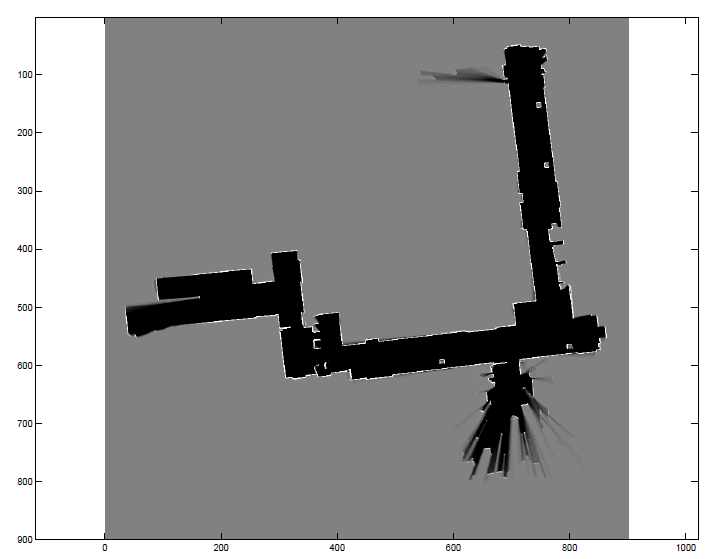o

### osc_ogi0qclx#### 暂无文章

hbase2.1.9 centos7 完全分布式 搭建随记

osc_4tfw1dxv
7分钟前
5
0
zookeeper3.5.5 centos7 完全分布式 搭建随记

osc_6jhxf9ab
9分钟前
7
0
steam夏日促销悄然开始，用Python爬取排行榜上的游戏打折信息

osc_ur9mmbck
10分钟前
5
0
python 里 certifi 库的作用

python 里 certifi 库的作用 安装了certifi之后，和requests库一样也有一个cacert.pem，可以用编辑器打开cacert.pem，里面包含了很多可信任知名公司的证书/公钥 库的路径，我这里是python2.7...

osc_1x6ycmfm
11分钟前
6
0

20大进阶架构专题每日送达 　　链接：yq.aliyun.com/articles/601745 　　2020年Java面试题库连载中 　　！ 　　正文 　　站在未来的路口，回望历史的迷途，常常会很有意思，因为我们会不...

osc_q5m9dzk0
13分钟前
13
0